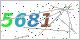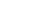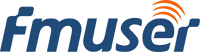Hot tag

Popular search

Recommended for You

# How To Distinguish DB, DBI, And DBM ? | FMUSER BROADCAST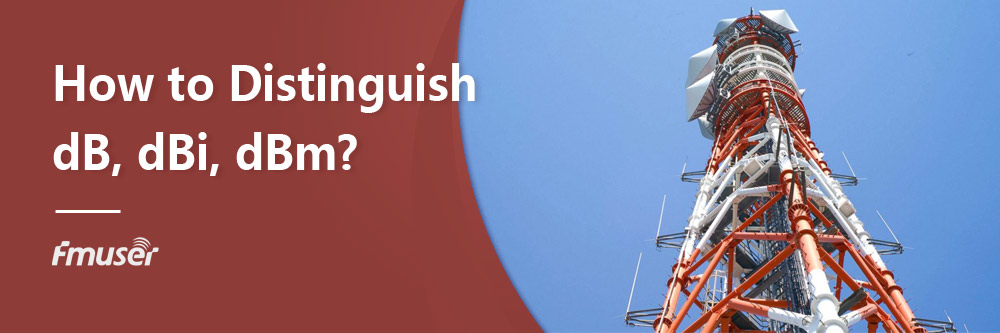If you have worked in the radio broadcasting industry for a period of time, you must have seen these units marked on some manuals of some radio broadcasting equipment like FM antenna or RF amplifier: dB, DBI, DBM. They look similar, but do you know what they mean and how to distinguish them? After reading this article, you will know their meaning and how to distinguish them.

Content

What is Gain?

How to Define and Distinguish dB, dBi, and dBm

Conclusion

The Definition of Gain

Before getting to the point, let's answer two questions: what does the gain of a FM broadcasting antenna mean ?

Based on Wikipedia, in a transmitting antenna, the gain describes how well the antenna converts input power into radio waves headed in a specified direction. In a receiving antenna, the gain describes how well the antenna converts radio waves arriving from a specified direction into electrical power. When no direction is specified, the gain is understood to refer to the peak value of the gain, the gain in the direction of the antenna's main lobe.

In short, the FM antenna can not improve the power of the transmitting device or the receiving device itself, but the antenna can concentrate these power or radio waves in a specified direction. In this way, the radio wave intensity emitted by the antenna in this direction will be stronger than the original one, which also means that the radio wave intensity in other directions will be weaker than the original one. So the gain is the ratio of the radio wave intensity in the direction with the strongest radiation intensity to the original radio wave intensity.

Back to Content

dB, dBi and dBm | Definition and Differences

After having a basic understanding of the concept of gain, it is much easier to understand the three units of dB, dBi, and dBm.

The Definition of dB

We learned in school that dB represents the loudness of sound. However, it is different in the RF field. Its formula is dB=10log(x/y)(where x and Y represent the radiation intensity of the two antennas) and represents the difference between the power levels of the two antennas(gain or loss)

Via calculating, we can know that if x is weaker than y, dB is negative; When x and y are equal, dB is equal to 0; When x = 2y, dB is equal to 3. Similarly, 6dB means x is equal to 4 times y and 12dB means x is equal to 16 times y. It should be noted that if you want to measure the actual gain or actual power difference of the wired antenna, please consider the loss of the RF cable itself.

The Definition of dBi

If you want to compare the radiation intensity of the gain directional antenna and omnidirectional antenna, you should take dBi as the unit, where "i" represents isotopic, and the calculation formula of dBi is the same as that of dBi

Because the omnidirectional antenna will radiate the radio signal with a perfect "sphere", that is, it has the same radio intensity in each direction. When the antenna has a gain in a specified direction, its lobe will narrow, that is, the FM radio station antenna takes a certain angle as the main radiation direction, and the radiation intensity is stronger than the original radiation intensity. The ratio of the radiation intensity of this main radiation angle to the original radiation intensity is the gain of this directional antenna. Therefore, when DBI is larger than 0, it indicates that the antenna has directivity.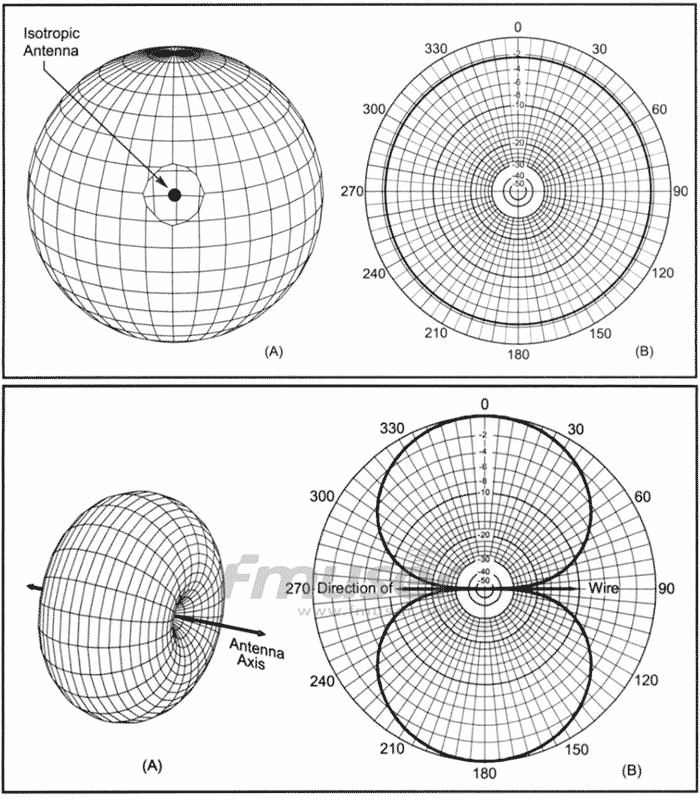The definition of dBm

Although dBm looks like dBi, it does not represent the radiation intensity. The "m" in dBm represents milliwatts (MW), which is similar to dBi, it is also a relative value, but it represents the relative value of transmission power with 1MW as the reference value. The formula is: dBm = 10 log (P1/1MW)

Although dBm is a relative value, it can be converted into the actual power of the equipment after unit conversion. For example, 0dbm = 1MW, 1dbm = 1.3MW, 10dBm = 1W, 60dbm = 1000W... It can be seen that it can use very simple values to represent very small power or very large power. Therefore, the actual power of various devices will be expressed in dBm.

The Differences among dB, dBi and dBm

In summary, dB, dBm, and dBm all are relative values, but they have the following 2 differences:

1. DB and DBI are used to represent the relative intensity (gain or loss) of the radio radiation of the antenna, while DBM is used to represent the actual power of the equipment.
2. DB is the relative value of the radiation intensity difference between two antennas, and DBI is the radio signal strength comparison of an antenna before and after gain (or orientation).

Back to Content

Back to Content

INQUIRY